# 3.1 Geometric Models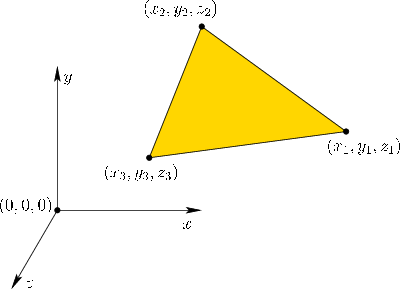We first need a virtual world to contain the geometric models. For our purposes, it is enough to have a 3D Euclidean space with Cartesian coordinates. Therefore, let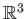denote the virtual world, in which every point is represented as a triple of real-valued coordinates: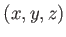. The coordinate axes of our virtual world are shown in Figure 3.1. We will consistently use right-handed coordinate systems in this book because they represent the predominant choice throughout physics and engineering; however, left-handed systems appear in some places, with the most notable being Microsoft's DirectX graphical rendering library. In these cases, one of the three axes points in the opposite direction in comparison to its direction in a right-handed system. This inconsistency can lead to hours of madness when writing software; therefore, be aware of the differences and their required conversions if you mix software or models that use both. If possible, avoid mixing right-handed and left-handed systems altogether.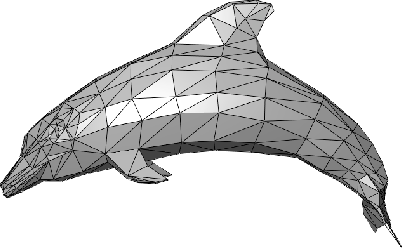Geometric models are made of surfaces or solid regions inand contain an infinite number of points. Because representations in a computer must be finite, models are defined in terms of primitives in which each represents an infinite set of points. The simplest and most useful primitive is a 3D triangle, as shown in Figure 3.1. A planar surface patch that corresponds to all points inside'' and on the boundary of the triangle is fully specified by the coordinates of the triangle vertices: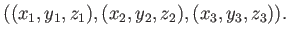(3.1)

To model a complicated object or body in the virtual world, numerous triangles can be arranged into a mesh, as shown in Figure 3.2. This provokes many important questions:

1. How do we specify how each triangle looks'' whenever viewed by a user in VR?
2. How do we make the object move''?
3. If the object surface is sharply curved, then should we use curved primitives, rather than trying to approximate the curved object with tiny triangular patches?
4. Is the interior of the object part of the model, or does the object consist only of its surface?
5. Is there an efficient algorithm for determining which triangles are adjacent to a given triangle along the surface?
6. Should we avoid duplicating vertex coordinates that are common to many neighboring triangles?
We address these questions in reverse order.

Subsections
Steven M LaValle 2019-03-14